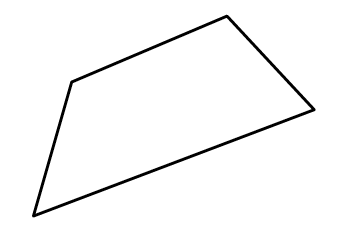### Home > CCAA > Chapter 4 Unit 3 > Lesson CC2: 4.2.4 > Problem4-67

4-67.

Alexa is looking at the shape at right. She needs to make several different figures that are similar to it.

1. If she uses the scale factor $\frac { 8 } { 5 }$, will the new shape that she creates be larger or smaller than the original? Justify your answer.

When multiplying by a number larger than $1$, will the new number be larger or smaller than it originally was? Consider multipliers in terms of scale factors.

2. List two different scale factors Alexa could use to make a smaller shape.

Any number less than $1$, but greater than $0$.

3. List two different scale factors Alexa could use to make a larger shape.

Any number greater than $1$.Explore the problem with the eTool below.
Click the link at right for the full version of the eTool:  (Desmos)